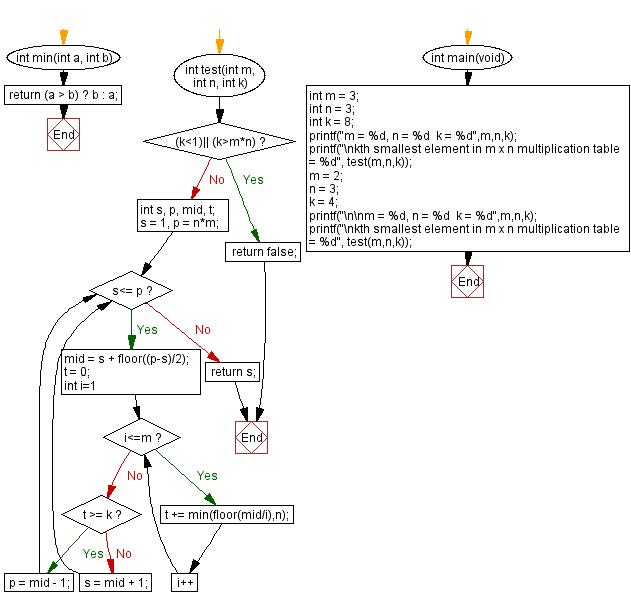﻿ C - Kth smallest number in a multiplication table

# C Programming: Kth smallest number in a multiplication table

## C Programming Mathematics: Exercise-34 with Solution

Write a C program that creates a multiplication table of size m x n using integers where 1 <= k <= m * n. Return the kth smallest element in the said multiplication table.

In mathematics, a multiplication table is a mathematical table used to define a multiplication operation for an algebraic system. The decimal multiplication table was traditionally taught as an essential part of elementary arithmetic around the world, as it lays the foundation for arithmetic operations with base-ten numbers.

Test Data:
(3,3,8) -> 6
(2,3,4) -> 3

Sample Solution:

C Code:

``````#include <stdio.h>
#include <math.h>

int min(int a, int b)
{
return (a > b) ? b : a;
}
int test(int m, int n, int k) {
if ((k<1)|| (k>m*n))
return false;
int s, p, mid, t;
s = 1, p = n*m;
while (s<= p) {
mid = s + floor((p-s)/2);
t = 0;
for (int i=1;i<=m;i++) {
t += min(floor(mid/i),n);
}

if (t >= k) {
p = mid - 1;
}
else {
s = mid + 1;
}
}
return s;
}
int main(void) {
int m = 3;
int n = 3;
int k = 8;
printf("m = %d, n = %d  k = %d",m,n,k);
printf("\nkth smallest element in m x n multiplication table = %d", test(m,n,k));
m = 2;
n = 3;
k = 4;
printf("\n\nm = %d, n = %d  k = %d",m,n,k);
printf("\nkth smallest element in m x n multiplication table = %d", test(m,n,k));
}

```
```

Sample Output:

```m = 3, n = 3  k = 8
kth smallest element in m x n multiplication table = 6
m = 2, n = 3  k = 4
kth smallest element in m x n multiplication table = 3

```

Flowchart:C Programming Code Editor:

Improve this sample solution and post your code through Disqus.

What is the difficulty level of this exercise?

Test your Programming skills with w3resource's quiz.

﻿